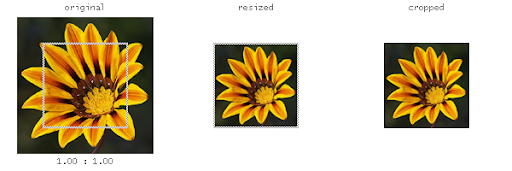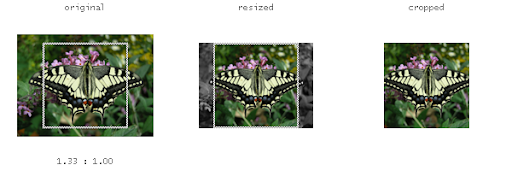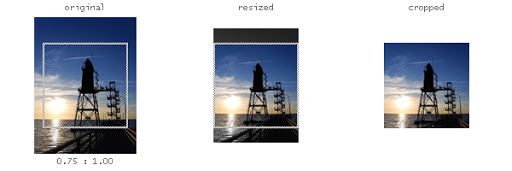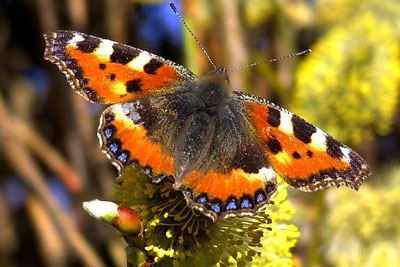# Crop-To-Fit an Image Using ASP/PHP

Image Cropping refers to the removal of the outer parts of an image to improve framing, accentuate subject matter or change aspect ratio. In this article I will demonstrate a technique that combines resizing and cropping to fit an image in given dimensions. You can, for example, use this technique to generate square thumbnails for images with arbitrary dimensions and aspect-ratio.

The following diagrams demonstrate what I am trying to accomplish here. Source images having arbitrary dimensions and aspect ratio are intelligently resized to desired dimensions and aspect ratio without distortion.

#### Example 1: When aspect ratios are same#### Example 2: When source image is wider#### Example 3: When source image is tallerThis is accomplished by resizing the image such that:

1. the original aspect ratio is maintained
2. one of the dimensions is equal to the desired size
3. the other dimension is equal to or greater than the desired size

Once resized, it is fairly straight-forward to calculate the cropping parameters.

#### ASP (Classic) Implementation

Unfortunately, classical ASP does not provide native methods for handling file uploads and processing images. Therefore, we will have to rely on server components. The example will demonstrate how you can utilize the following two commercially available components for this task:

1. Persits Software AspUpload
2. Persits Software AspJpeg
##### The HTML Form

The HTML form posts the file to the processing script. You may notice the use of `enctype="multipart/form-data"` in the following form.

```<form action="image-crop-demo.asp" method="post" enctype="multipart/form-data">
Upload an image for processing<br>
<input type="file" name="Image1"><br>
</form>```
##### The ASP Code
```<%@ language="vbscript" %>
<%
option explicit

'----------------------------------------------------------------
' Crop-to-fit ASPJPEG
' Revision 2 [2009-06-01]
' Corrected aspect ratio of the output image
'----------------------------------------------------------------

const DESIRED_IMAGE_WIDTH = 150
const DESIRED_IMAGE_HEIGHT = 150

'
' Ideally, you should set the properties for ASPUPLOAD component here
'

dim oFile
set oFile = oUpload.Files( "Image1" )

dim oJpeg
set oJpeg = Server.CreateObject( "PERSITS.JPEG" )
'
' Ideally, you should set the properties for PERSITS.JPEG component here
'
'
' This example requires that PreserveAspectRatio is set to FALSE
' Otherwise you may notice round-off errors when ASPJPEG resizes the image
'
oJpeg.PreserveAspectRatio = false

oJpeg.OpenBinary( oFile.Binary )

dim SourceAspectRatio
dim DesiredAspectRatio

SourceAspectRatio = oJpeg.Width / oJpeg.Height
DesiredAspectRatio = DESIRED_IMAGE_WIDTH / DESIRED_IMAGE_HEIGHT

if SourceAspectRatio > DesiredAspectRatio then
'
' Triggered when source image is wider
'
oJpeg.Height = DESIRED_IMAGE_HEIGHT
oJpeg.Width = DESIRED_IMAGE_HEIGHT * SourceAspectRatio
else
'
' Triggered otherwise (i.e. source image is similar or taller)
'
oJpeg.Width = DESIRED_IMAGE_WIDTH
oJpeg.Height = DESIRED_IMAGE_WIDTH / SourceAspectRatio
end if

dim X0
dim Y0
dim X1
dim Y1
'
' Crop the image keeping the CENTER part intact
'
X0 = ( oJpeg.Width - DESIRED_IMAGE_WIDTH ) / 2
Y0 = ( oJpeg.Height - DESIRED_IMAGE_HEIGHT ) / 2
X1 = X0 + DESIRED_IMAGE_WIDTH
Y1 = Y0 + DESIRED_IMAGE_HEIGHT

oJpeg.Crop X0, Y0, X1, Y1

oJpeg.SendBinary
%>```

#### PHP+GD Library Implementation

The code differs from the resize image using php and gd library example I posted earlier. The main difference is in the way the resize dimensions are calculated. And then there is an additional step that crops the resized image.

##### The HTML Form

The HTML form remains exactly the same as above except the action attribute.

```<form action="image-crop-demo.php" method="post" enctype="multipart/form-data">
Upload an image for processing<br>
<input type="file" name="Image1"><br>
</form>```
##### The PHP Code
```<?php
//----------------------------------------------------------------
// Crop-to-fit PHP-GD
// Revision 2 [2009-06-01]
// Corrected aspect ratio of the output image
//----------------------------------------------------------------

define( 'DESIRED_IMAGE_WIDTH', 150 );
define( 'DESIRED_IMAGE_HEIGHT', 150 );

\$source_path = \$_FILES[ 'Image1' ][ 'tmp_name' ];

//
// Add file validation code here
//

list( \$source_width, \$source_height, \$source_type ) = getimagesize( \$source_path );

switch ( \$source_type )
{
case IMAGETYPE_GIF:
\$source_gdim = imagecreatefromgif( \$source_path );
break;

case IMAGETYPE_JPEG:
\$source_gdim = imagecreatefromjpeg( \$source_path );
break;

case IMAGETYPE_PNG:
\$source_gdim = imagecreatefrompng( \$source_path );
break;
}

\$source_aspect_ratio = \$source_width / \$source_height;
\$desired_aspect_ratio = DESIRED_IMAGE_WIDTH / DESIRED_IMAGE_HEIGHT;

if ( \$source_aspect_ratio > \$desired_aspect_ratio )
{
//
// Triggered when source image is wider
//
\$temp_height = DESIRED_IMAGE_HEIGHT;
\$temp_width = ( int ) ( DESIRED_IMAGE_HEIGHT * \$source_aspect_ratio );
}
else
{
//
// Triggered otherwise (i.e. source image is similar or taller)
//
\$temp_width = DESIRED_IMAGE_WIDTH;
\$temp_height = ( int ) ( DESIRED_IMAGE_WIDTH / \$source_aspect_ratio );
}

//
// Resize the image into a temporary GD image
//

\$temp_gdim = imagecreatetruecolor( \$temp_width, \$temp_height );
imagecopyresampled(
\$temp_gdim,
\$source_gdim,
0, 0,
0, 0,
\$temp_width, \$temp_height,
\$source_width, \$source_height
);

//
// Copy cropped region from temporary image into the desired GD image
//

\$x0 = ( \$temp_width - DESIRED_IMAGE_WIDTH ) / 2;
\$y0 = ( \$temp_height - DESIRED_IMAGE_HEIGHT ) / 2;

\$desired_gdim = imagecreatetruecolor( DESIRED_IMAGE_WIDTH, DESIRED_IMAGE_HEIGHT );
imagecopy(
\$desired_gdim,
\$temp_gdim,
0, 0,
\$x0, \$y0,
DESIRED_IMAGE_WIDTH, DESIRED_IMAGE_HEIGHT
);

//
// Render the image
// Alternatively, you can save the image in file-system or database
//

header( 'Content-type: image/jpeg' );
imagejpeg( \$desired_gdim );

//
// Add clean-up code here
//
?>```

#### Example Output

Original ImageASPJPEG OutputPHP+GD Output